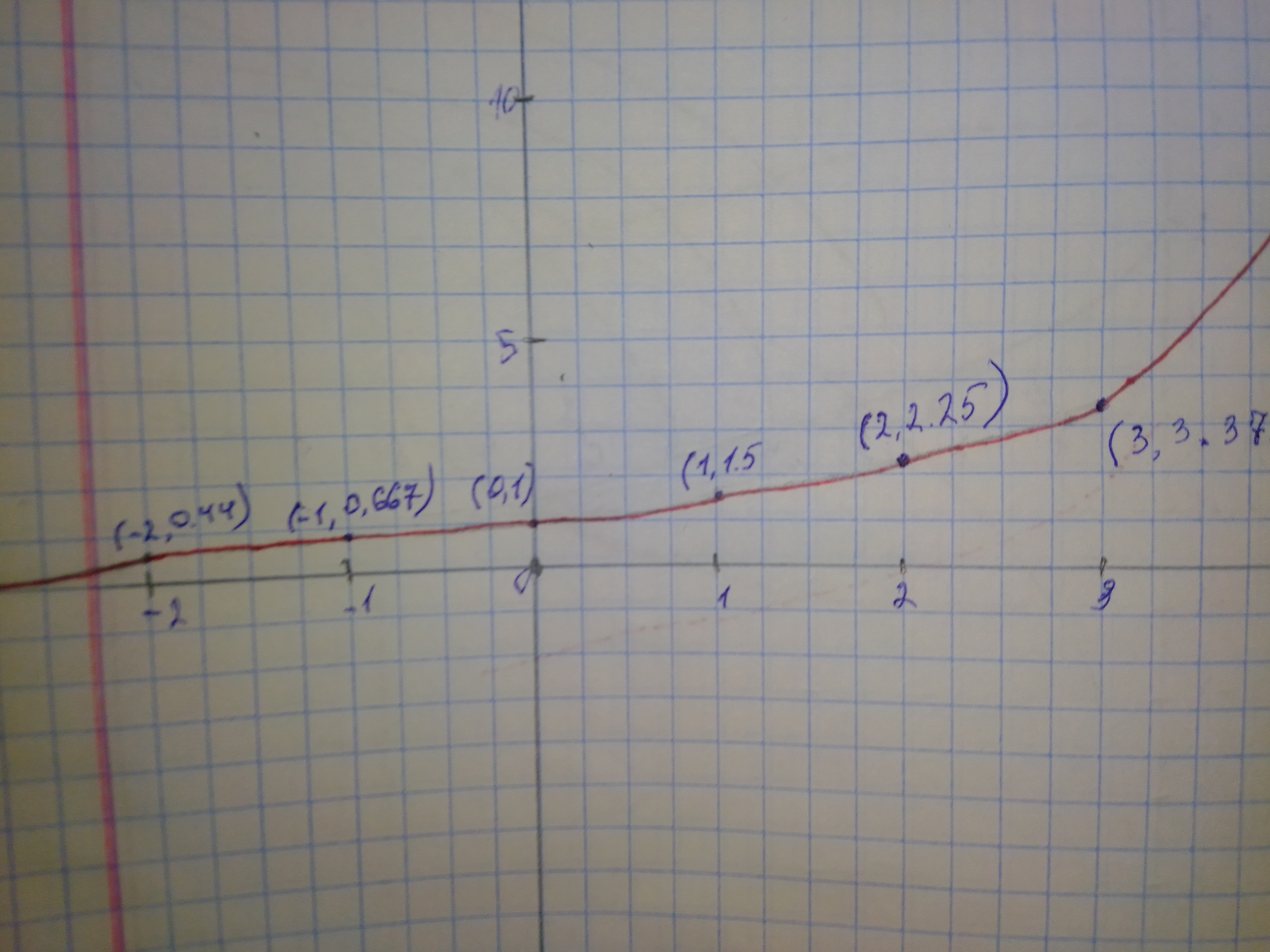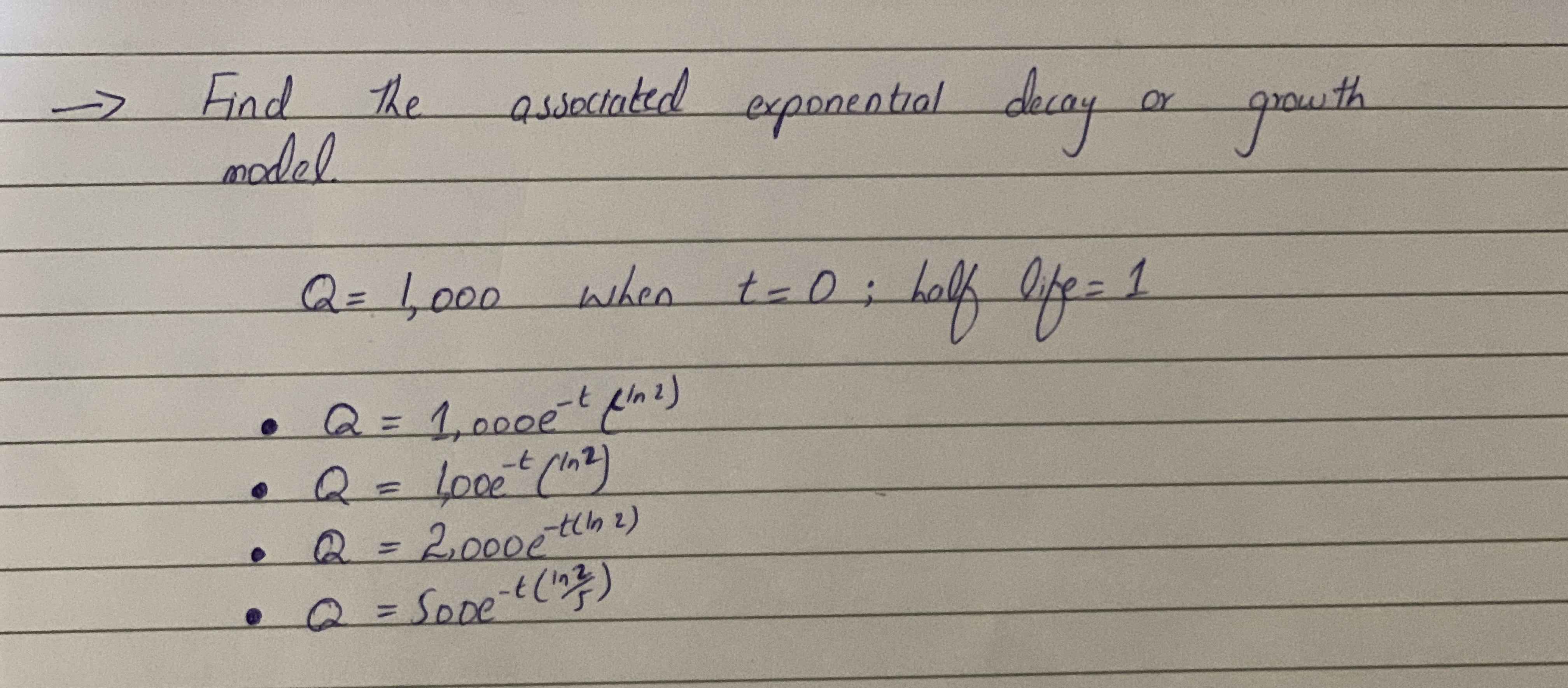Ask question

# Tell whether the function represents exponential growth or exponential decay. Then graph the function. f(x)=(1.5)^{x}# Tell whether the function represents exponential growth or exponential decay. Then graph the function. f(x)=(1.5)^{x}

Question
Exponential growth and decayasked 2020-12-12

Tell whether the function represents exponential growth or exponential decay. Then graph the function. $$f(x)=(1.5)^{x}$$

## Answers (1)2020-12-13

Step 1 Given: $$y=1.5^{x}$$

Step 2 \begin{array}{|c|c|} \hline x & -2 & -1 & 0 & 1 & 2 & 3\\ \hline y & 0.444 & 0.666 & 1 & 1.5 & 2.25 & 3.375 \\ \hline \end{array} Step 3### Relevant Questionsasked 2021-02-05

Tell whether the function represents exponential growth or decay. $$h(x)=2.5(0.8)^x$$asked 2021-05-01

Determine whether the function represents exponential growth or exponential decay. Identify the percent rate of change. Then graph the function. $$y=5^x$$asked 2021-03-06

Determine whether each equation represents exponential growth or exponential decay. Find the rate of increase or decrease for each model. Graph each equation. $$y=5^x$$asked 2021-02-04

State whether the equation represents exponential growth, exponential decay, or neither. $$y=0.9^x$$asked 2021-01-31

For the following exercises, determine whether the equation represents exponential growth, exponential decay, or neither. Explain. $$y=11,701(0.97)^t$$asked 2021-03-06

Decide if the equation represents exponential growth or decay. Explain your answer. $$y=(3^{x})^{2}$$asked 2021-01-27

A bacteria population is growing exponentially with a growth factor of $$\frac{1}{6}$$ each hour.By what growth rate factor does the population change each half hour.Select all that apply
a: $$\frac{1}{12}$$
b: $$\sqrt{\frac{1}{6}}$$
c: $$1/3$$
d: $$\sqrt6$$
e: $$\frac{1}{6}^{\frac{1}{2}}$$asked 2021-02-01.asked 2021-02-11

Tell whether the function represents exponential growth or exponential decay. Then graph the function. $$f(x)=(0.25)^{x}$$asked 2021-05-29
Determine whether each function represents exponential growth or exponential decay. Identify the percent rate of change.
$$\displaystyle{g{{\left({t}\right)}}}={2}{\left({\frac{{{5}}}{{{4}}}}\right)}^{{t}}$$
...